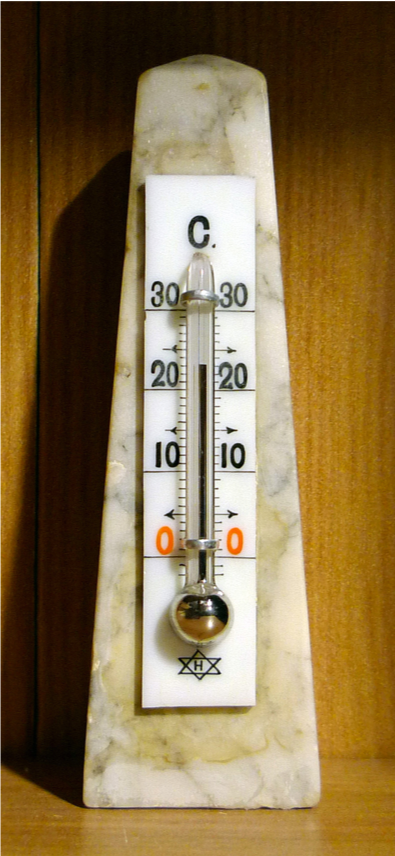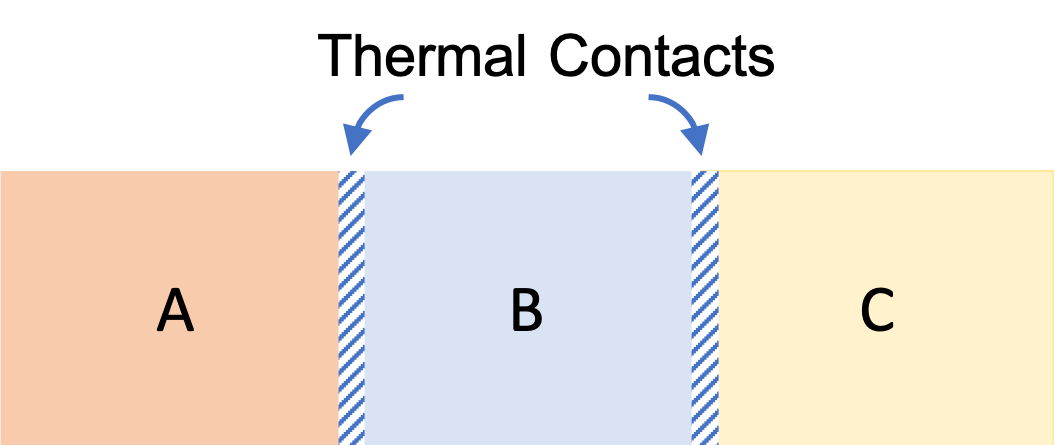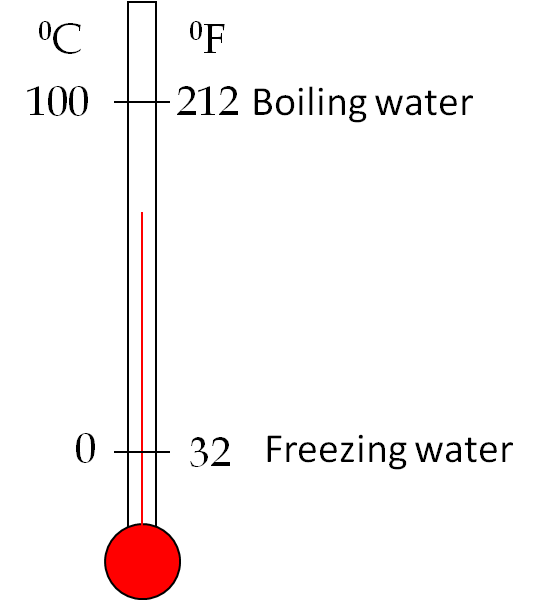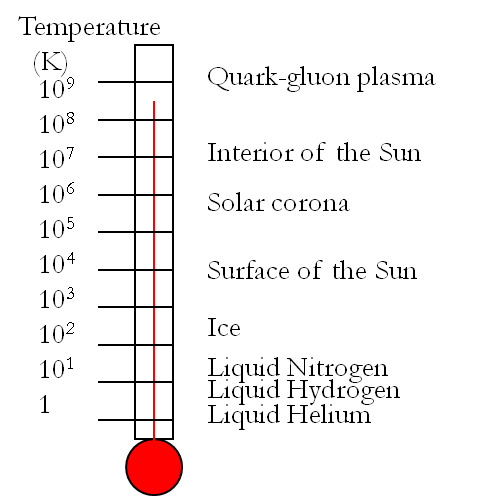## Section19.3Temperature

Most people will say that a body at a higher temperature feels warmer than the body at a lower temperature. Although this is true if we touch samples made of same material, our sense of touch is not a good indicator of temperature if we are comparing temperatures of different substances. For instance, a metal at room temperature feels colder than wood at the same temperature since metal conducts away heat from our body more quickly. Similarly, an alumnimum foil in the oven will feel much warmer, it may even burn you, compared to glass in the same oven.

An objective indicator of temperature would be any physical property that changes with temperature. Various properties are used for this purpose. You may be familiar with mercury thermometers (the picture on the right, credit: Wikimedia, Anominski), which depends on the expansion of volume of mercury when it is heated. Any property that changes with temperature, can be used to build instruments, called thermometers, that can provide quantitative measure of temperature. For some situations, mercury thermometer is not suitable. A thermocouple is widely used for temperature; it uses voltage between two metals as temperature indicator.### Subsection19.3.1Thermal Equilibrium

Temperature is actually a property associated with the state of thermal equilibrium. Suppose you place a wooden block, initially at room temperature, into an oven whose temperature is set at $300^{\circ}\text{F}\text{.}$ After some time has passed, you will notice that the wooden block's temperature is also $300^{\circ}\text{F}\text{.}$ We say that the system (the block) has reached in thermal equilibrium with the environment (the oven) due to the fact that wooden block was not insulated from the oven, but could exchange heat with it.

Generally, all systems that are in thermal contact with each other will reach thermal equilibrium with every system having the same temperature.

One important consequence of the definition of temperature in terms of the thermal equilibrium is that if a system is in thermal equilibrium internally, the temperature at all points inside the system will be the same. When this happens we say that the system is at a particular temperature. If a system is not in thermal equilibrium internally, then the system would have different temperatures at different points inside the system. For instance, when you heat water on a hot plate, the water near the plate is usually at a higher temperature than the water at points further away.

### Subsection19.3.2Zeroth Law of Thermodynamics

The zeroth law of thermodynamics states that if a system A is in thermal equilibrium with a system B, which, in turn, is in thermal equilibrium with another system C, then systems A and C are also in thermal equilibrium with each other regardless of whether or not A and C are in direct contact.Figure 19.3.1. Thermal contact between systems permits the three systems to cme to thermal equilibrium which is characterized by the three systems reaching the same temperature.

Thus all systems at the same temperature can be thought to be in thermal equilibrium with each other, or equivalently, all systems in thermal equilibrium must have the same temperature. If two systems, which are not in thermal contact but have the same temperature, are brought in thermal contact, then there will be no heat transfer between them.

### Subsection19.3.3Temperature Scales

To make temperature a more quantitative property we need an operational definition of temperature which we can implement with real materials. In particular, we need to find reproducible thermal equilibrium situations, to which numerical values of temperature may be assigned, and track a property that varies with temperature as a conduit of the value of temperature.

Historically, freezing and boiling points of water at atmospheric pressure were selected as highly reproducible equilibrium states of matter. The two common scales of temperature, the Celsius or centigrade (C) and Fahrenheit (F), use these reference states.

In the Celsius scale, we assign a value of zero degree to the freezing point of water and a value of 100 degrees to the boiling point of water. In the Fahrenheit scale, the freezing point is assigned 32 degrees and the boiling point 212 degrees. There are 180 degrees in Fahrenheit scale between the freezing and boiling points of water while there are only 100 degrees in the Celsius scale.Therefore, each degree change in the Celsius scale is equal to $\dfrac{180}{100}$ or $\dfrac{9}{5}$ degrees change in the Fahrenheit scale. If a particular temperature reads C degrees in Celsius and F degrees in Fahrenheit, then their relation will be:

\begin{equation} F = \dfrac{9}{5}\ C + 32.\tag{19.3.1} \end{equation}

In scientific work, another temperature scale, called the Absolute or kelvin scale is often used, especially in low-temperature physics or cryogenics. This scale has a strong theoretical basis with claims that zero temperature in this scale may never be breached. A reading in kelvin scale to be denoted in this book by the capital letter $T$ is related to the Celsius scale reading $\text{C}$ for the same condition as follows.

\begin{equation} T = C + 273.15.\tag{19.3.2} \end{equation}

Since 1954, the temperature scale has been fixed by first assigning a temperature of 273.16 degrees to the triple point of water in the Absolute or kelvin (K) temperature scale. The triple point is defined as the state when all three phases of water, namely ice, liquid water and vapor are in equilibrium at atmospheric pressure, i.e., a mixture of iced water with vapor above it. We assign it a value of $273.16K\text{.}$

\begin{equation} \text{Temperature of the triple point of water}\ = 273.16K.\tag{19.3.3} \end{equation}

We assign temperature for other conditions by the process described in Subsection 19.3.4 below.

In nature we find a wide range of temperature as shown in the figure. the temperature of the universe as seen in the background radiation is approximately $2.7\text{K}$ and $\gt 10\text{ MK}$ inside stars. In laboratories physicists have been able to push the temperatures to as low as $100\text{ pK}$ in a piece of rhodium metal and as high as $1\text{ GK}$ in quark-gluon plasma.### Subsection19.3.4Calibration Using The Triple Point of Water

Since 1954 the triple point of water, a state in which a mixture of ice, liquid water and vapor coexist in equilibrium, has been chosen as the standard reference which is arbitrarily assigned a value of 273.16 K.

Let $X$ be a property that we use for the thermometer, e.g., the volume of a mercury thermometer. Let the value of $X$ at the triple point be $X = X_\text{TP}\text{.}$ Then, a thermometer that is in equilibrium with triple point water will have

\begin{equation} 273.16 = a X_\text{TP},\label{eq-thermometric-triple-1}\tag{19.3.4} \end{equation}

for some value of $a\text{.}$ Now, we want to know the temperature of something else. Suppose when we place the thermometer in contact with the unknown, we find that value of $X=X_\text{T}\text{,}$ then

\begin{equation} T = a X_\text{T}.\label{eq-thermometric-triple-2}\tag{19.3.5} \end{equation}

Dividing this equation by (19.3.4) we remove the unknown constant $a$ and obtain the value of the unknown temperature from measured value of X.

\begin{equation} T = \left(\dfrac{273.16\ K}{X_\text{TP}} \right) X_\text{T}\tag{19.3.6} \end{equation}

### Subsection19.3.5Change in Temperature in Different Scales

Many thermodynamic properties are stated as per unit change in temperature. For instance, we would like to know how much length changes if we change the temperature by $1^{\circ}\text{C}$ versus by $1^{\circ}\text{F}\text{.}$ They are not the same change.

Let $\Delta C$ be a change in temperature stated in the Celsius scale, and similarly $\Delta F$ for Fahrenheit and $\Delta T$ in kelvin scale. Their relation will be

\begin{align} \amp \Delta C = \Delta T,\tag{19.3.7}\\ \amp \Delta F = \dfrac{9}{5}\ \Delta C. \tag{19.3.8} \end{align}

On a fine day, the temperature in San Francisco was $68^{\circ}\text{F}\text{.}$ What are the readings in Celsius and kelvin scales?

Hint

Use Celsius/Fahrenheit formula

$20^{\circ}\text{C}\text{,}$ $293.15\text{K}\text{.}$

Solution

From the relation between Fahrenheit and Celsius scales we get

\begin{equation*} t(^{\circ}\text{C}) = \dfrac{5}{9} \left( t(^{\circ}\text{F})-32 \right) = 20^{\circ}\text{C}. \end{equation*}

From Celsius reading we can get the kelvin scale reading very simply by adding $273.15\text{.}$

\begin{equation*} t(\text{K}) = t(^{\circ}\text{C}) + 273.15 = 293.15\text{K}. \end{equation*}

Suppose the normal temperature of your body is $98^{\circ}\text{F}\text{.}$ After jogging a mile, you find that your temperature has gone up to $99^{\circ}\text{F}\text{.}$

(a) What is your normal body temperature in Celsius and Kelvin scales?

(b) By how much has your temperature gone up in Celsius and Kelvin scales?

Hint

(a) Use Celsius/Fahrenheit formula, (b) Use deltas.

(a) $36.7^{\circ}\text{C},\ 309.9\text{K}\text{,}$ (b) $0.56^{\circ}\text{C},\ 0.56\text{K} \text{.}$

Solution 1 (a)

(a) Finding the equivalent reading in Celsius scale requires a direct application of the formula relating Celsius and Fahrenheit given above.

\begin{equation*} F=\dfrac{9}{5}C + 32 \Longrightarrow C =\dfrac{5}{9} (F-32) = 36.7^{\circ}\text{C} \end{equation*}

The reading T in Kelvin scale is obtained by simply adding $273.15$ to the Celsius reading.

\begin{equation*} T = 36.7+273.15 = 309.9\ \text{K} \end{equation*}
Solution 2 (b)

(b) From the relation between the Celsius and Fahrenheit

\begin{equation*} F=\dfrac{9}{5}C + 32 \end{equation*}

we see that the rise in Fahrenheit is $\dfrac{9}{5}$ times the rise in Celsius. Note the adjustment of $32^{\circ}\text{F}$ for $0^{\circ}\text{C}$ would not appear in the difference in temperature readings.

\begin{align*} \amp \Delta F = \dfrac{9}{5}\Delta C \\ \amp \Longrightarrow \Delta C = \dfrac{5}{9}\Delta F =\dfrac{5}{9}\times 1=0.56^{\circ}\text{C}. \end{align*}

The rise in Kelvin $\Delta T$ is same as the rise in Celsius. Therefore,

\begin{equation*} \Delta T =\Delta C = 0.56^{\circ}\text{C} = 0.56\text{K}. \end{equation*}

The temperature on a nice day in Missouri, USA is $65^{\circ}\text{F}\text{.}$ What will be the readings in the Celsius and Kelvin scales?

Hint

Use conversion formulas.

$18.3^{\circ}\text{C}\text{,}$ $291.45\text{K}\text{.}$

Solution
\begin{align*} C \amp = \dfrac{5}{9}(F - 32) = \dfrac{5}{9}(65 - 32) = 18.3^{\circ}.\\ K \amp = C + 273.15 = 18.3 + 273.15 = 291.45^{\circ}. \end{align*}

The temperature on a hot day in Kolkata, India is $42^{\circ}\text{C}\text{.}$ What will be the readings in the Fahrenheit and Kelvin scales?

Hint

Use conversion formulas.

$107.6^{\circ}\text{F}\text{,}$ $315.15\text{K}\text{.}$

Solution
\begin{align*} F \amp = \dfrac{9}{5}C + 32 = \dfrac{9}{5}\times 42+ 32 = 107.6^{\circ}.\\ K \amp = C + 273.15 = 42 + 273.15 = 315.15^{\circ}. \end{align*}

In an experiment, the temperature of a copper plate is reported to change from $375.00\text{K}$ to $375.25\text{K}\text{.}$ How would you describe the change in temperature in Celsius and Fahrenheit scales?

Hint

The additive constant part cancels out when you convert difference in temperature.

$0.25^\circ\text{C},\ 0.45^\circ\text{F}$